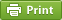﻿ Lewis structure of C2H6:Biochemhelp

# Lewis structure of C2H6## Step method to draw lewis structure of ethane

Step 1: Find valence e- for all atoms. Add them together.

C:4x2=8
H=1x6=6

Total=14

Step2: Find octet e- for each atom and add them together.

C:8x2=16
H:2x6=12

Total=28

Chlorine gets 10 electrons in order to make 3 bonds with surrounding atoms.

Step3: Gives you bonding e-. Subtract step 1 total from step 2

28-14=14e-

Step 4: Find number of bonds by diving the number in step 3 by 2(because each bond is made of 2 e-)

14e-/2= 7 bond pairs

Step 5: Find the number of nonbonding (lone pairs) e-. Subtract step 3 number from step 1.

14-14= 0 e-=0 lone pairs

Use information from step 4 and 5 to draw the lewis structure.## Lewis dot structure of C2H6

Alternatively a dot method can be used to draw the lewis structure.
Calculate the total valence electrons in the molecule.
C:4x2=8
H=1x6=6

Total=14

Put two carbons in the center and arrange hydrogena toms on the sides.Arrange electrons until both carbons get 8 electrons.